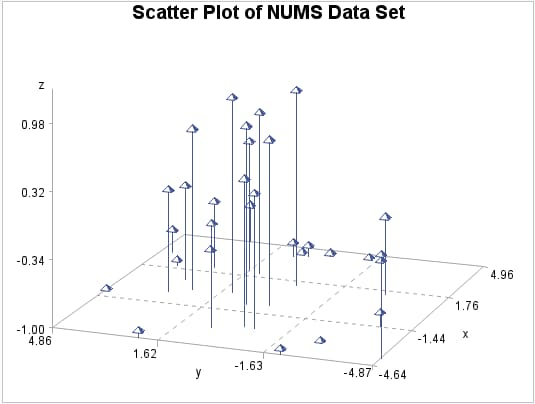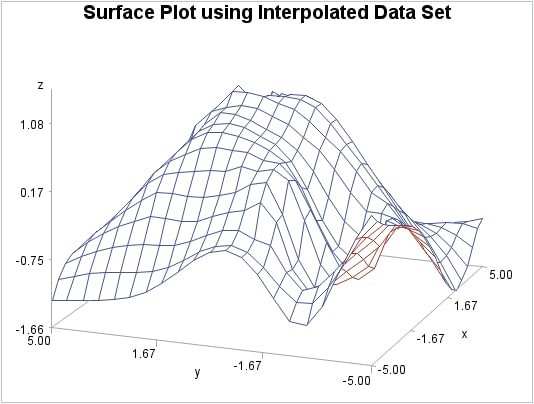# Overview: G3GRID Procedure

The G3GRID procedure processes an existing SAS data set to create a data set that the G3D procedure or the GCONTOUR procedure can use to produce a three-dimensional surface plot or a contour plot. The procedure creates a data set whose horizontal X-Y variable values form a complete grid, and it interpolates the values of the vertical Z variable for each point on the X-Y plane.
Using the G3GRID procedure, you can do the following actions:
• create a rectangular grid of interpolated or smoothed values from irregularly spaced observations for use in a three-dimensional surface or contour plot
• complete a rectangular grid of interpolated or smoothed values for an input data set that has an insufficient number of observations to produce a three-dimensional surface or contour plot
• interpolate or smooth data for a three-dimensional plot
The G3GRID procedure does not produce graphics output. PROC G3GRID produces an output data set that you can use as the input data set for PROC G3D or PROC GCONTOUR.
Surface Plot of Data Set after G3GRID Processing (gtdefin) illustrate the effect of the G3GRID procedure on data.
This figure shows a collection of data points, where z=f(x,y). These points are randomly distributed, and cannot be displayed with a G3D surface plot, although they can be displayed with a scatter plot.
Scatter Plot of Data Set before G3GRID Processing (gtgdefin)The following figure shows a surface plot of the data set that is created by a G3GRID interpolation of the original data set shown in the preceding figure. The evenly distributed horizontal (x,y) data points form a grid for the three-dimensional plot.
Surface Plot of Data Set after G3GRID Processing (gtdefin)